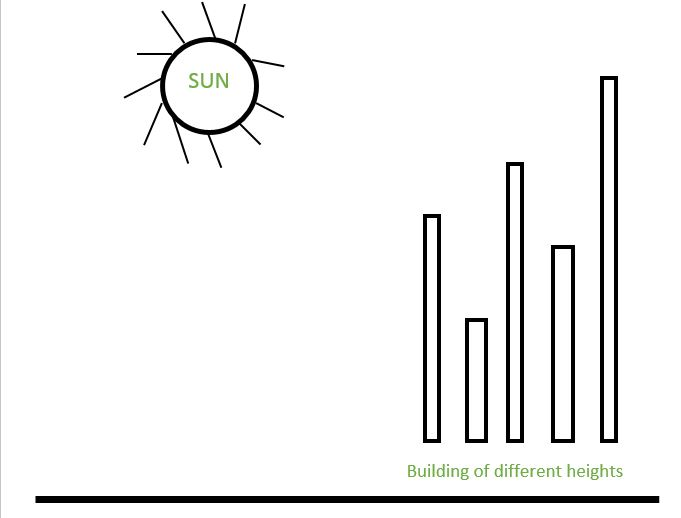GeeksforGeeks App
Open AppBrowser
Continue

# Number of buildings facing the sun

Given an array representing heights of buildings. The array has buildings from left to right as shown in below diagram, count number of buildings facing the sunset. It is assumed that heights of all buildings are distinct.Examples:

```Input : arr[] = {7, 4, 8, 2, 9}
Output: 3
Explanation: As 7 is the first element, it can
see the sunset.
4 can't see the sunset as 7 is hiding it.
8 can see.
2 can't see the sunset.
9 also can see the sunset.

Input : arr[] = {2, 3, 4, 5}
Output : 4```

It can be easily observed that only the maximum element found so far will see the sunlight
i.e. curr_max will see the sunlight and then only the element greater than curr_max will see the sunlight. We traverse given array from left to right. We keep track of maximum element seen so far. Whenever an element becomes more than current max, increment result and update current max.

Implementation:

## C++

 `// C++ program to count buildings that can``// see sunlight.``#include ``using` `namespace` `std;` `// Returns count buildings``// that can see sunlight``int` `countBuildings(``int` `arr[], ``int` `n)``{``    ``// Initialize result (Note that first building``    ``// always sees sunlight)``    ``int` `count = 1;` `    ``// Start traversing element``    ``int` `curr_max = arr;``    ``for` `(``int` `i = 1; i < n; i++) {``      ` `        ``// If curr_element is maximum``        ``// or current element is``        ``// equal, update maximum and increment count``        ``if` `(arr[i] > curr_max || arr[i] == curr_max) {``            ``count++;``            ``curr_max = arr[i];``        ``}``    ``}` `    ``return` `count;``}` `// Driver code``int` `main()``{``    ``int` `arr[] = { 7, 4, 8, 2, 9 };``    ``int` `n = ``sizeof``(arr) / ``sizeof``(arr);``    ``cout << countBuildings(arr, n);``    ``return` `0;``}`

## Java

 `// Java program to count buildings that can``// see sunlight.` `class` `Test {``    ``// Returns count buildings that can see sunlight``    ``static` `int` `countBuildings(``int` `arr[], ``int` `n)``    ``{``        ``// Initialize result  (Note that first building``        ``// always sees sunlight)``        ``int` `count = ``1``;` `        ``// Start traversing element``        ``int` `curr_max = arr[``0``];``        ``for` `(``int` `i = ``1``; i < n; i++) {``          ` `            ``// If curr_element is maximum``            ``// or current element``            ``// is equal, update maximum and increment count``            ``if` `(arr[i] > curr_max || arr[i] == curr_max) {``                ``count++;``                ``curr_max = arr[i];``            ``}``        ``}` `        ``return` `count;``    ``}` `    ``// Driver method``    ``public` `static` `void` `main(String[] args)``    ``{``        ``int` `arr[] = { ``7``, ``4``, ``8``, ``2``, ``9` `};` `        ``System.out.println(countBuildings(arr, arr.length));``    ``}``}`

## Python3

 `# Python3 program to count buildings``# that can see sunlight.` `# Returns count buildings that``# can see sunlight`  `def` `countBuildings(arr, n):` `    ``# Initialize result (Note that first``    ``# building always sees sunlight)``    ``count ``=` `1` `    ``# Start traversing element``    ``curr_max ``=` `arr[``0``]``    ``for` `i ``in` `range``(``1``, n):` `        ``# If curr_element is maximum or``        ``# current element is equal,``        ``# update maximum and increment count``        ``if` `(arr[i] > curr_max ``or` `arr[i] ``=``=` `curr_max):` `            ``count ``+``=` `1``            ``curr_max ``=` `arr[i]` `    ``return` `count`  `# Driver code``arr ``=` `[``7``, ``4``, ``8``, ``2``, ``9``]``n ``=` `len``(arr)``print``(countBuildings(arr, n))` `# This code is contributed by Rohit.`

## C#

 `// C# program to count buildings that can``// see sunlight.``using` `System;` `class` `GFG {``  ` `    ``// Returns count buildings``    ``// that can see sunlight``    ``static` `int` `countBuildings(``int``[] arr, ``int` `n)``    ``{``        ` `        ``// Initialize result (Note that first building``        ``// always sees sunlight)``        ``int` `count = 1;` `        ``// Start traversing element``        ``int` `curr_max = arr;``        ``for` `(``int` `i = 1; i < n; i++) {``          ` `            ``// If curr_element is maximum``            ``// or current element``            ``// is equal, update maximum``            ``// and increment count``            ``if` `(arr[i] > curr_max || arr[i] == curr_max) {``                ``count++;``                ``curr_max = arr[i];``            ``}``        ``}` `        ``return` `count;``    ``}` `    ``// Driver method``    ``public` `static` `void` `Main()``    ``{``        ``int``[] arr = { 7, 4, 8, 2, 9 };` `        ``Console.Write(countBuildings(arr, arr.Length));``    ``}``}` `// This code is contributed by Rohit.`

## PHP

 ` ``\$curr_max` `|| ``\$arr``[``\$i``] == ``\$curr_max``)``        ``{``            ``\$count``++;``            ``\$curr_max``=``\$arr``[``\$i``];``        ``}``    ``}` `    ``return` `\$count``;``}` `// Driver code``\$arr` `= ``array``(7, 4, 8, 2, 9);``\$n` `= sizeof(``\$arr``) / sizeof(``\$arr``);``echo` `countBuildings(``\$arr``, ``\$n``);` `// This code is contributed by``// Rohit``?>`

## Javascript

 ``

Output

`3`

Time Complexity: O(n)
Auxiliary Space: O(1)

This article is contributed by Sahil Chhabra. If you like GeeksforGeeks and would like to contribute, you can also write an article using write.geeksforgeeks.org or mail your article to review-team@geeksforgeeks.org. See your article appearing on the GeeksforGeeks main page and help other Geeks.

My Personal Notes arrow_drop_up